# Bestselling Pharmaceutical Drugs

Let’s see how we can visualize the Best selling pharmaceuticals of 2017/18 using PPTXHandler.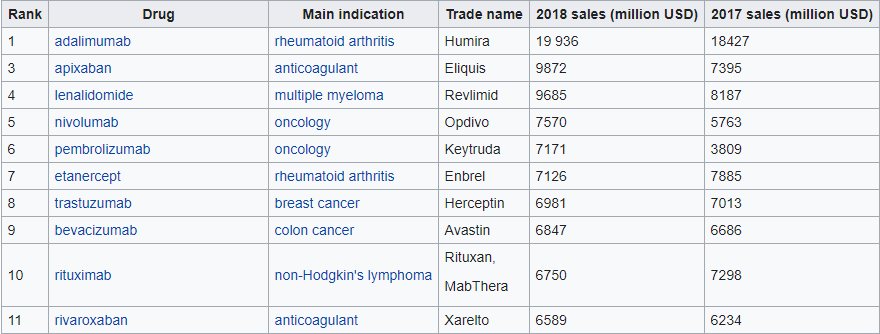We’re aiming for a result like this: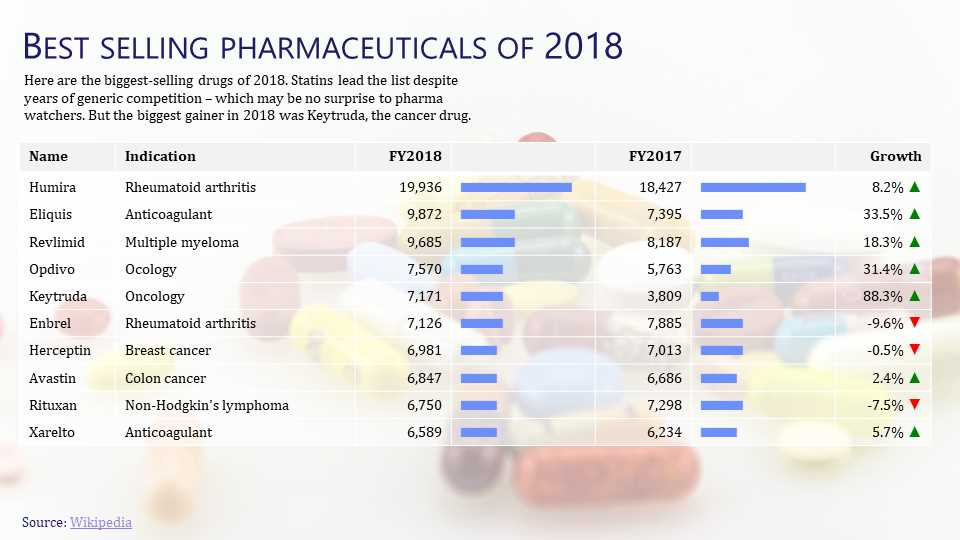## Create the source template

Start by creating a template.pptx that has the static content laid out.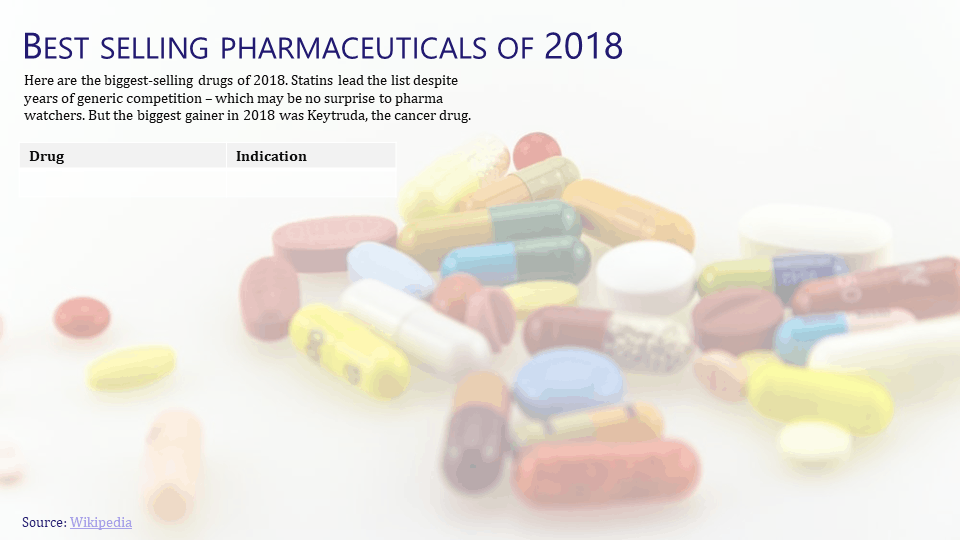All of the content is static, except for the table called `Sales Table`.

## Create the data

The data is available from the Wikipedia. Let’s copy the data into a CSV file sales.csv.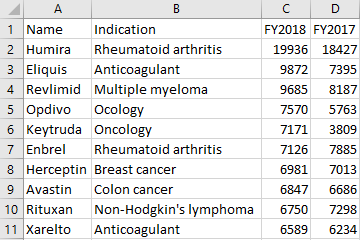## Create the rules

Let’s set up a basic `gramex.yaml` file that loads the template and the data.

``````url:
pptxhandler/table:
pattern: /\$YAMLURL/output.pptx
handler: PPTXHandler
kwargs:
version: 2
source: \$YAMLPATH/template.pptx
data:
sales:
url: \$YAMLPATH/sales.csv
``````

The data has fewer columns than we need, so let’s add 3 columns:

• `Bar 2018`: a dummy column to show the bar chart of 2018 sales
• `Bar 2017`: a dummy column to show the bar chart of 2017 sales
• `Growth`: Yearly growth in 2018

We add these columns with the Pandas `transform:` key. This needs a single expression, so we combine the transforms using `or`.

``````          transform: >
data.insert(3, 'Bar 2018', data['FY2018']) or
data.insert(5, 'Bar 2017', data['FY2017']) or
data.insert(6, 'Growth', data['FY2018'] / data['FY2017'] - 1) or
data
``````

We now set up the basic formatting for the table, using `sales` data. This also sets the font size and vertical alignment for the entire table.

``````      rules:
- Sales Table:
table:
data: sales
vertical-align: f'middle'
font-size: f'11 pt'
``````

That gives us this result.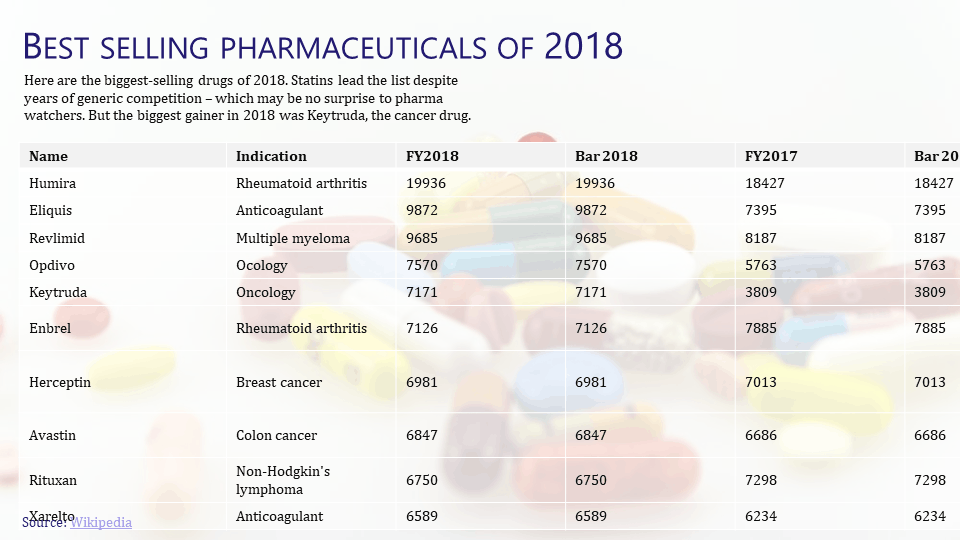Let’s start by adjusting the table headers, and aligning the numeric columns to the right.

``````              header-row:
- Name
- Indication
- '<p align="right">FY2018</p>'
- ''
- '<p align="right">FY2017</p>'
- ''
- '<p align="right">Growth</p>'
``````

Now, let’s look at the text. The first two columns are straight-forward.

``````              text:
Name: cell.val
Indication: cell.val
``````

For the numbers, we just need to right align them and add a comma separator (e.g. “10,000” instead of “10000”). We use Python string formatting for this.

``````                FY2018: f'<p align="right">{cell.val:,}</p>'
FY2017: f'<p align="right">{cell.val:,}</p>'
``````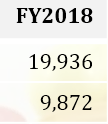For the bars, we repeat the same “█” character multiple times, using

• a blue shade (`accent_1` from the theme)
• Arial font
• 6 pt (determined through trial and error)

For every \$1,000 million (or a billion) of sales, we show one “█”.

``````                Bar 2018: f'<a color="accent_1" font-name="Arial" font-size="6pt">' + ('█' * int(cell.val / 1000)) + '</a>'
Bar 2017: f'<a color="accent_1" font-name="Arial" font-size="6pt">' + ('█' * int(cell.val / 1000)) + '</a>'
``````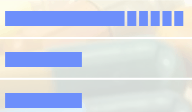We’ve manually added spaces between character to give you an idea of character width.

Finally, the Growth column has a red ▼ or a green ▲ depending on whether growth was positive or negative.

``````                Growth: f'<p align="right">{cell.val:.1%} ' +
('<a color="red">▼</a>' if cell.val < 0 else '<a color="green">▲</a>') +
'</p>'
``````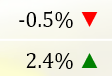Finally, let’s adjust the width of these columns (determined by trial and error).

``````              width:
Name: 1
Indication: 2.5
FY2018: 1
Bar 2018: 1.5
FY2017: 1
Bar 2017: 1.5
Growth: 1
``````

This is the final configuration

## See the result

This is the output PPTX